# NCERT Solutions For Class 10 Maths Chapter 1 Exercise 1.1 – Real Numbers

Download NCERT Solutions For Class 10 Maths Chapter 1 Exercise 1.1 – Real Numbers. This Exercise contains 5 questions, for which detailed answers have been provided in this note. In case you are looking at studying the remaining Exercise for Class 10 for Maths NCERT solutions for other Chapters, you can click the link at the end of this Note.### NCERT Solutions For Class 10 Maths Chapter 1 Exercise 1.1 – Real Numbers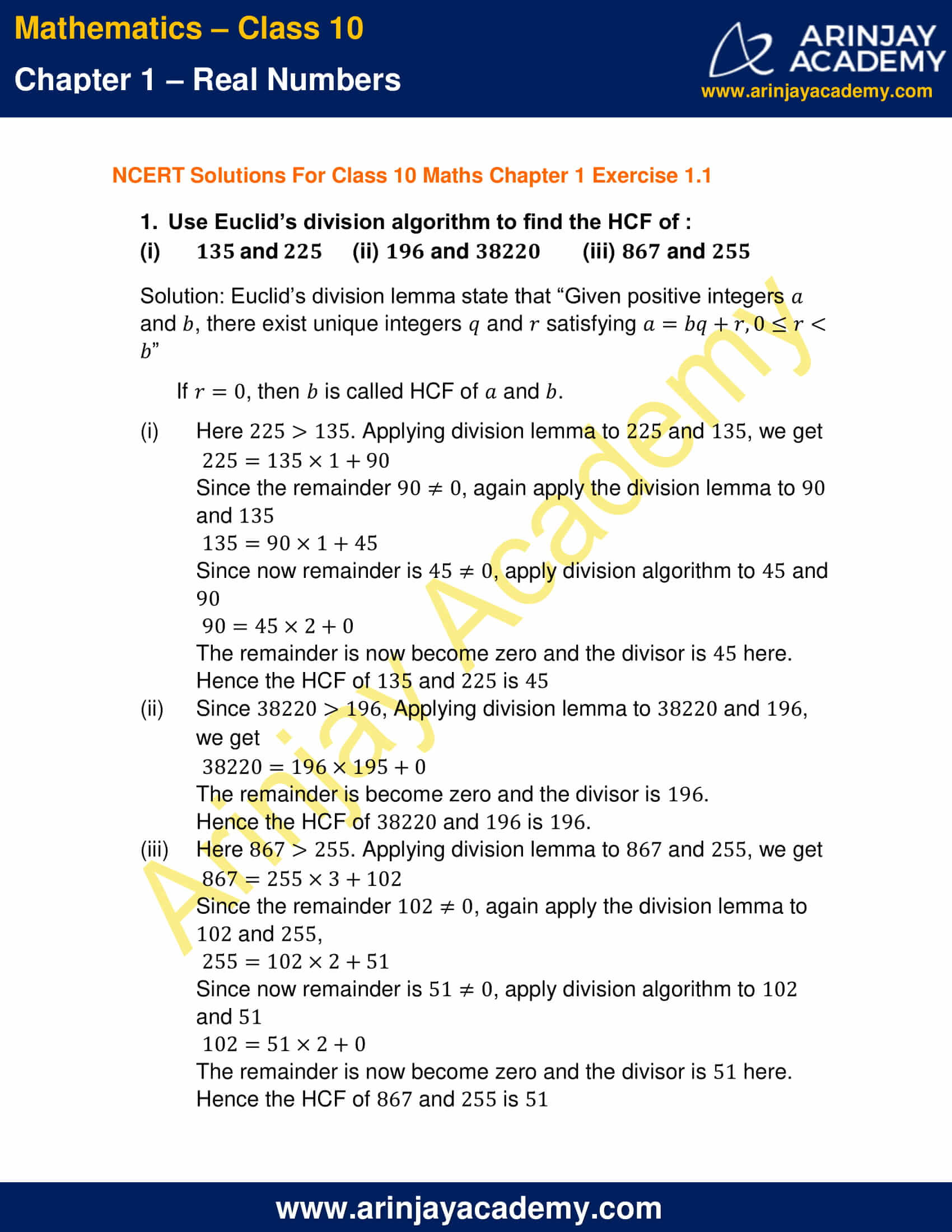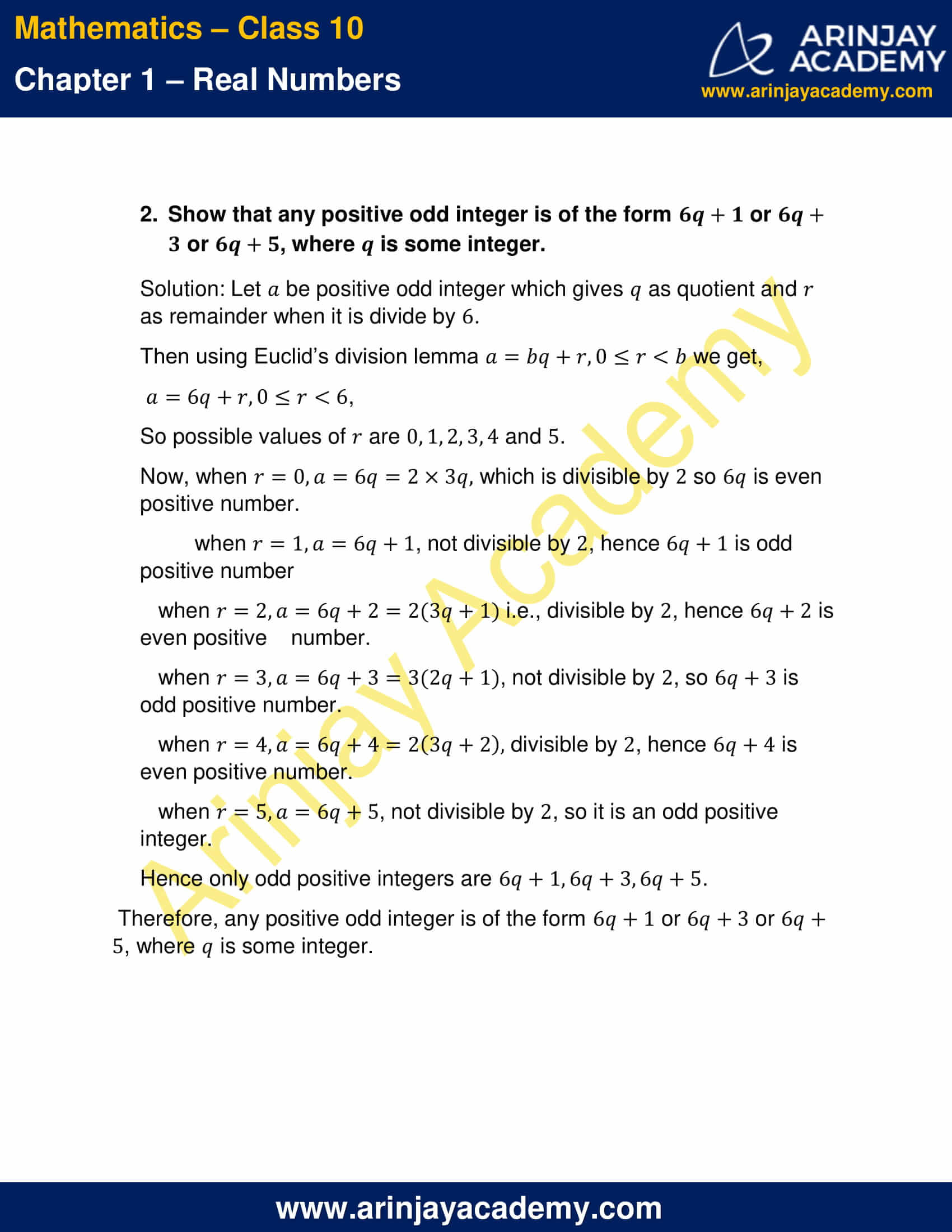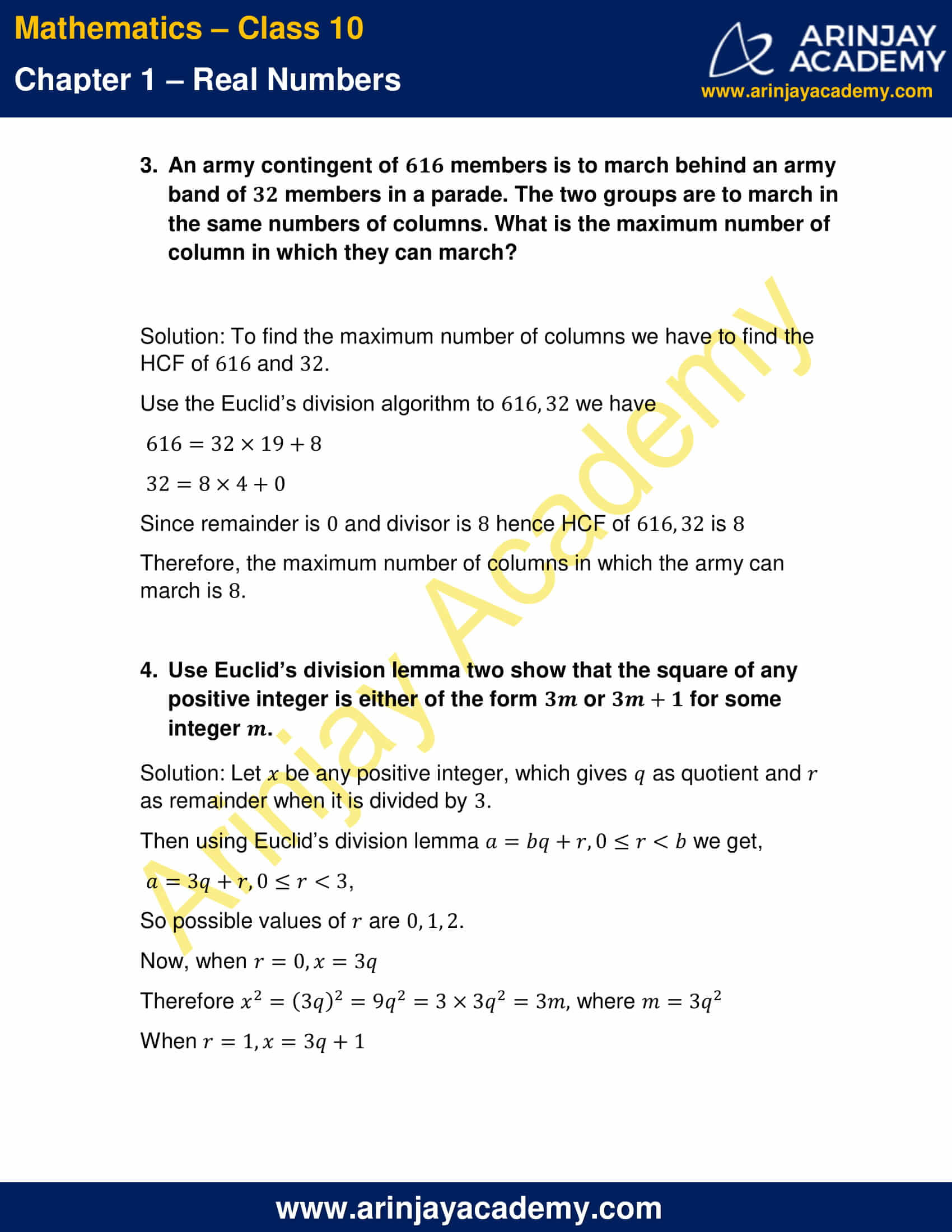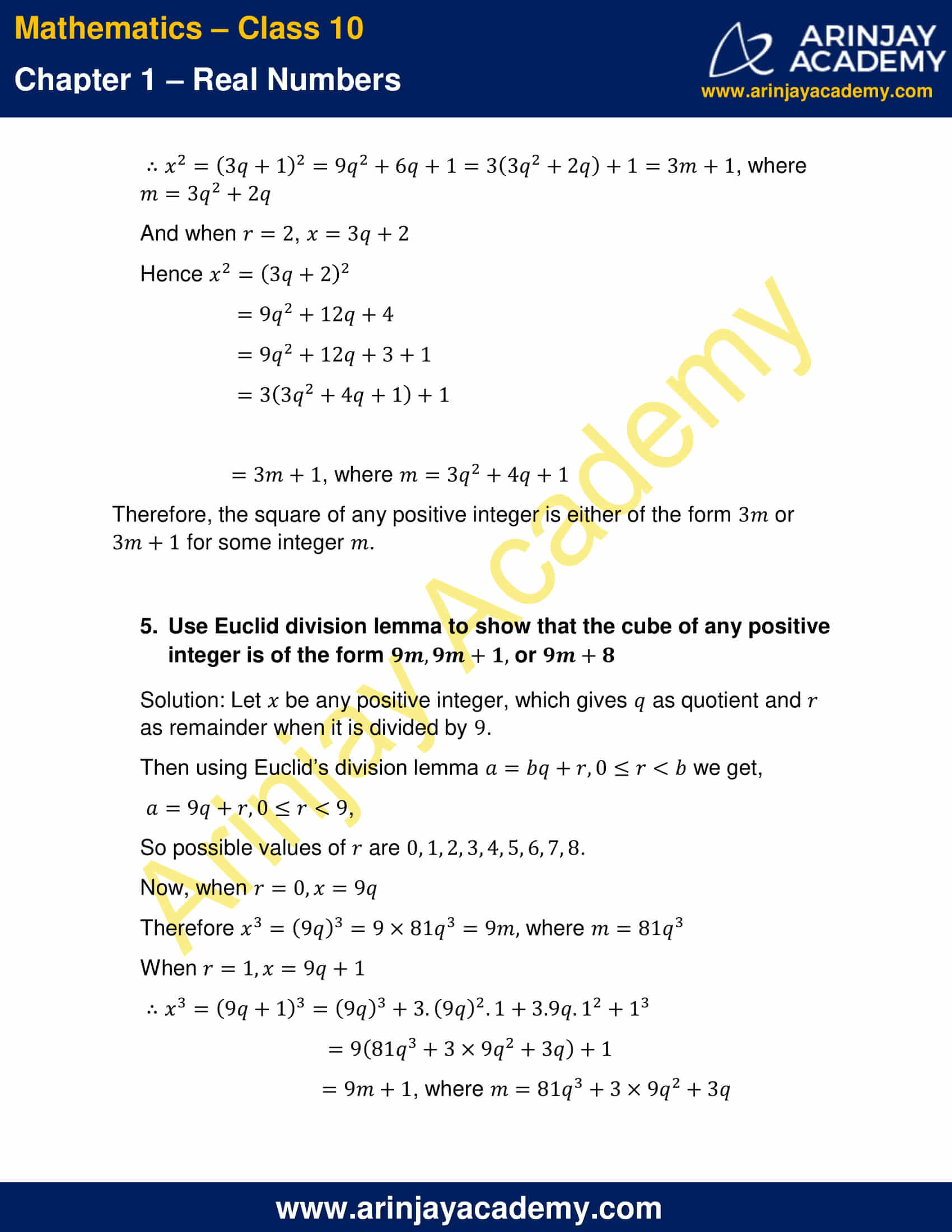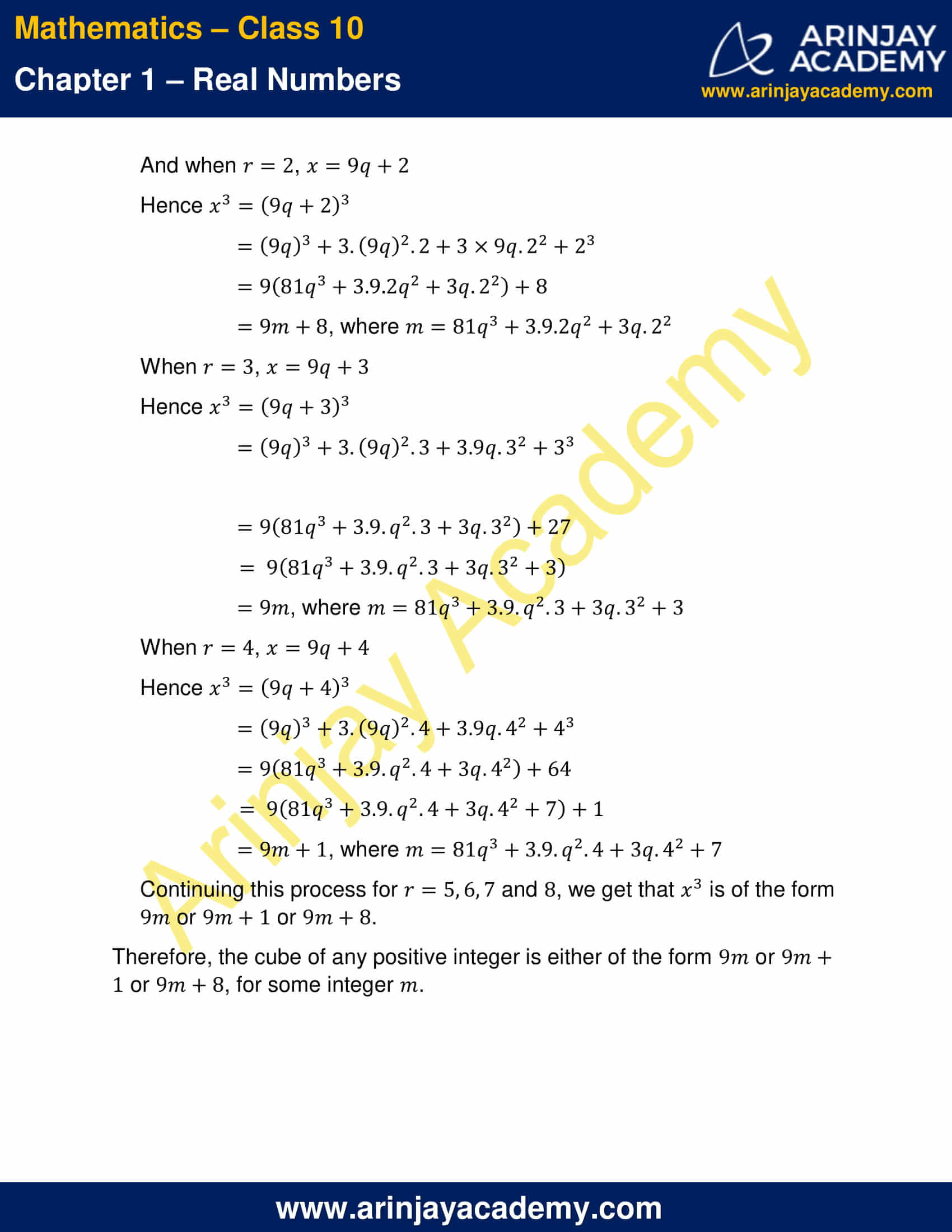NCERT Solutions For Class 10 Maths Chapter 1 Exercise 1.1 – Real Numbers

1. Use Euclid’s division algorithm to find the HCF of :

(i) 135 and 225
(ii) 196 and 38220
(iii) 867 and 255

Solution: Euclid’s division lemma state that “Given positive integers  and , there exist unique integers and satisfying  a = bq + r, 0≤r<b”
If , r = 0 then b, is called HCF of a and b.

(i) Here 225 > 135. Applying division lemma to 225 and 135, we get
225 = 135 x 1 + 90
Since the remainder 90 ≠ 0, again apply the division lemma to 90 and 135
135 = 90 x 1 + 45
Since now remainder is 45 ≠ 0, apply division algorithm to 45 and 90
90 = 45 x 2 + 0
The remainder is now become zero and the divisor is 45 here.
Hence the HCF of 135 and 225 is 45

(ii) Since 38220 > 196, Applying division lemma to 38220 and 196, we get
38220 = 196 x 195 + 0
The remainder is become zero and the divisor is 196.
Hence the HCF of 38220 and 196 is 196.

(iii) Here 867 > 255. Applying division lemma to 867 and 255, we get
867 = 255 x 3 + 102
Since the remainder 102 ≠ 0, again apply the division lemma to 102 and 255
255 = 102 x 2 + 51
Since now remainder is 51 ≠ 0, apply division algorithm to 102 and 51
102 = 51 x 2 + 0
The remainder is now become zero and the divisor is 51 here.
Hence the HCF of 867 and 255 is 51

2. Show that any positive odd integer is of the form 6q + 1 or 6q + 3 or 6q + 5, where q is some integer.

Solution: Let a be positive odd integer which gives q as quotient and r as remainder when it is divide by 6.
Then using Euclid’s division lemma a = bq + r, 0 ≤ r < b we get,
a = 6q + r, 0 ≤ r < 6,
So possible values of r are 0, 1, 2, 3, 4 and 5.
Now, when r = 0, a = 6q = 2 x 3q, which is divisible by 2 so 6q is even positive number.
when, r = 1, a = 6q + 1, not divisible by 2, hence 6q + 1 is odd positive number.
when, r = 2, a = 6q + 2 = 2(3q + 1 ) i.e., divisible by 2, hence 6q + 2 is even positive number.
when, r = 3, a = 6q + 3 = 3(2q + 1), not divisible by 2, so 6q + 3 is odd positive number.
when, r = 4, a = 6q + 4 = 2(3q + 2),  divisible by 2, hence 6q + 4 is even positive number.
when, r = 5, a = 6q + 5, not divisible by 2, so it is an odd positive integer.
Hence only odd positive integers are 6q + 1, 6q + 3, 6q + 5.
Therefore, any positive odd integer is of the form 6q + 1 or 6q + 3 or 6q + 5, where q is some integer.

3. An army contingent of 616 members is to march behind an army band of 32 members in a parade. The two groups are to march in the same numbers of columns. What is the maximum number of column in which they can march?

Solution: To find the maximum number of columns we have to find the HCF of 616 and 32.
Use the Euclid’s division algorithm to 616, 32 we have
616 = 32 x 19 + 8
32 = 8 x 4 + 0
Since remainder is 0 and divisor is 8 hence HCF of  is 616, 32 is 8
Therefore, the maximum number of columns in which the army can march is 8.

4. Use Euclid’s division lemma two show that the square of any positive integer is either of the form or  for some integer .

Solution: Let x be any positive integer, which gives q as quotient and r as remainder when it is divided by 3.
Then using Euclid’s division lemma a = bq + r, 0 ≤ r < b we get,
a = 3q + r, 0 ≤ r < 3,
So possible values of r are 0, 1, 2.
Now, when r = 0, x = 3q
Therefore, x2  = (3q)2  = 9q2 = 3 x 3q2 = 3m, where m= 3q2
When, r = 1, x = 3q + 1
x2 = (3q + 1)2 = 9q2 + 6q + 1 = 3(3q2 + 2q) + 1 = 3m + 1 where m = 3q2 + 2q
And when r = 2, x = 3q + 2
Hence x2 = (3q + 2)2
= 9q2 + 12q + 4
= 9q2 + 12q + 3 + 1
= 3(3q2 + 4q + 1) + 1
= 3m + 1, where m= 3q2 + 4q + 1) + 1
Therefore, the square of any positive integer is either of the form 3m or 3m + 1 for some integer m.

5. Use Euclid division lemma to show that the cube of any positive integer is of the form 9m, 9m + 1, or 9m + 8

Solution: Let x be any positive integer, which gives q as quotient and r as remainder when it is divided by 9.
Then using Euclid’s division lemma a = bq + r, 0 ≤ r < b we get,
a = 9q + r, 0 ≤ r < 9 we get,
So possible values of r are 0, 1, 2, 3, 4, 5, 6, 7, 8.

Now, when r = 0, x = 9q
Therefore, x3 = (9q)3 = 9 x 81q3 = 9m, where m = 81q3
When r = 1, x = 9q + 1
x3 = (9q + 1)3 = (9q)3 + 3.(9q)2.1 + 3.9q.12 + 13
9(81q3 + 3 x 9q2 + 3q) + 1
= 9m + 1, where m = 81q3 + 3 x 9q2 + 3q

When r = 2, x = 9q + 2
Hence x3 = (9q + 2)3
= (9q)3 + 3.(9q)2.2 + 3 x 9q.22 + 23
= 9(81q3 + 3.9.2q2 + 3q.22) + 8
= 9m + 8, where m = 81q3 + 3.9.2q2 + 3q.22

When r = 3, x = 9q + 3
Hence x3 = (9q + 3)3
= (9q)3 + 3.(9q)2.3 + 3.9q.32 + 33
= 9(81q3 + 3.9.q2.3 + 3q.32) + 27
= 9(81q3 + 3.9.q2.3 + 3q.32 + 3)
= 9m, where m = 81q3 + 3.9.q2.3 + 3q.32 + 3

When r = 4, x = 9q + 4
Hence x3 = (9q + 4)3
= (9q)3 + 3.(9q)2.4 + 3.9q.42 + 43
= 9(81q3 + 3.9.q2.4 + 3q.42) + 64
= 9(81q3 + 3.9.q2.4 + 3q.42 + 7) + 1
= 9m + 1, where m = 81q3 + 3.9.q2.4 + 3q.42 + 7

Continuing this process for r = 5, 6, 7 and 8, we get that x3 is of the form 9m or 9m + 1 or 9m + 8.
Therefore, the cube of any positive integer is either of the form 9m or 9m + 1 or 9m + 8, for some integer m.

NCERT Solutions for Class 10 Maths Chapter 1 Exercise 1.1 – Real Numbers, has been designed by the NCERT to test the knowledge of the student on the topic – Euclid’s Division Lemma

### 1 thought on “NCERT Solutions For Class 10 Maths Chapter 1 Exercise 1.1 – Real Numbers”

1.aweswome